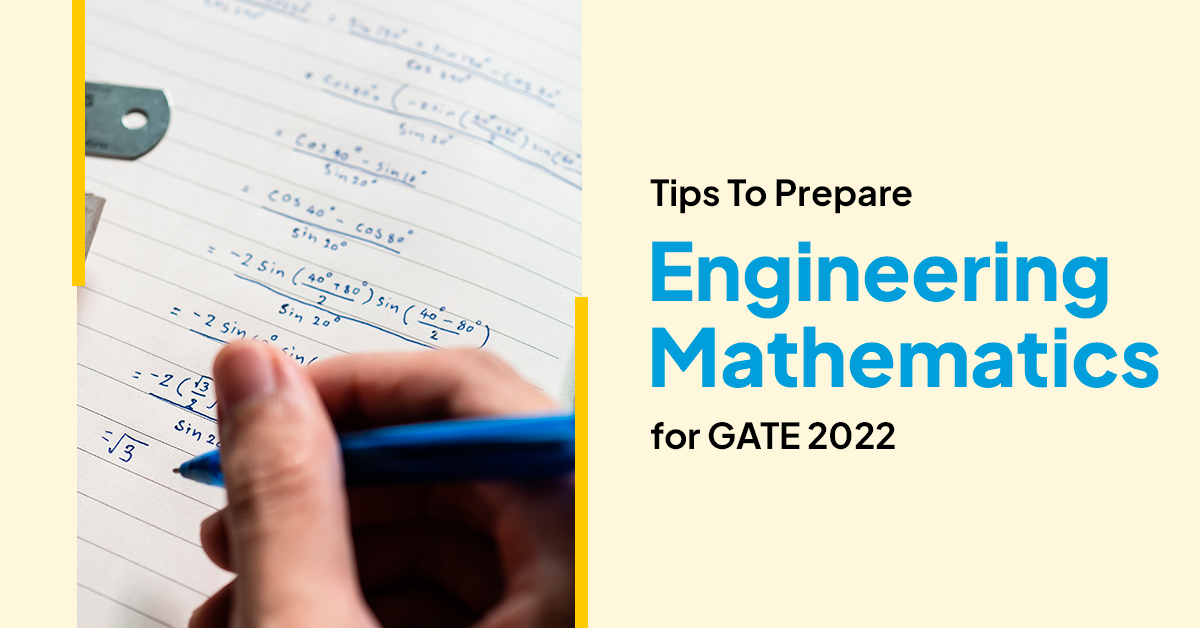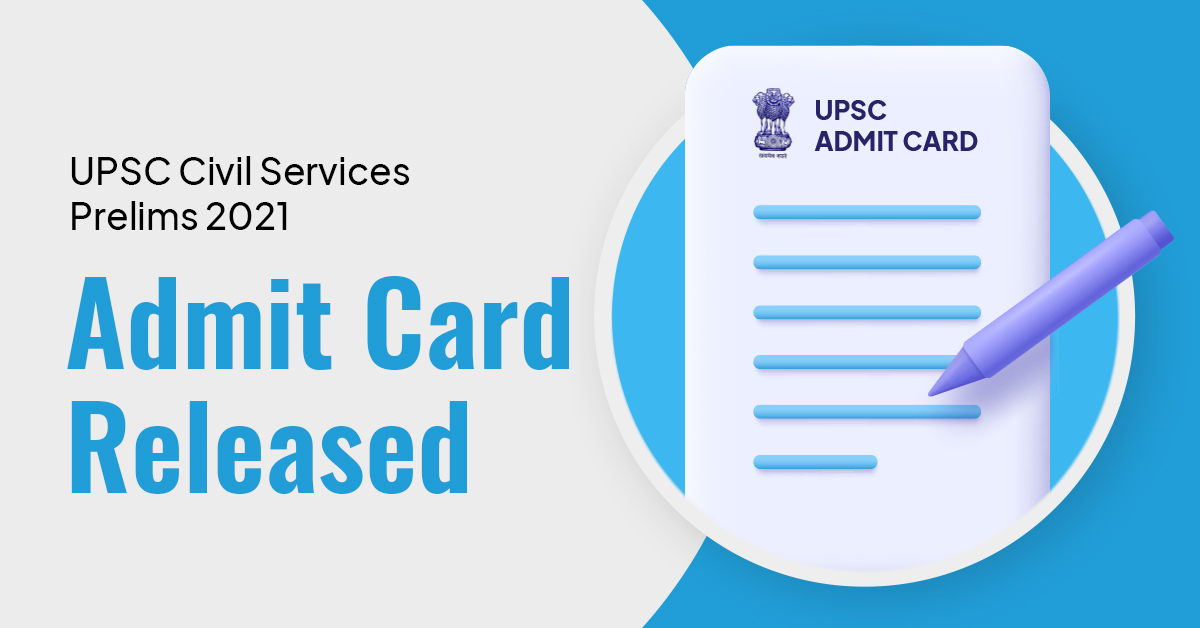## Tips To Prepare Engineering Mathematics For GATE 2022If you want to change your GATE Game, then Engineering Mathematics is the Game Changer!

Irrespective of any subject (Paper) you choose for the General Aptitude Test in Engineering (GATE), Engineering Mathematics is one of the compulsory subjects you have to appear. It is a scoring subject and has a high marks weightage, 13% of the total marks of GATE. The unique part is that the marks weightage has remained the same for the last many years. Hence, it deserves special attention in GATE exam preparation

With these crucial marks at stake, it becomes essential to strategically prepare for Engineering Mathematics, as performing well in this section can get you closer to your dream score.

Unlike high school mathematics, Engineering Mathematics at the GATE level is more advanced and has topics that require deep comprehension. Consider it as an extension of Class XI and XII Mathematics. Engineering Mathematics plays a significant role in GATE 2022 exam preparation, and taking it for granted will cost you crucial GATE marks.

In this article, we will share some practical tips that will help you prepare for Engineering Mathematics with ease. But before we begin, let’s look at the syllabus of Engineering Mathematics and some important topics.

Also read: How To Fill GATE 2022 Application Form?

## Engineering Mathematics GATE 2022 Syllabus & Important Topics

A detailed analysis of the Engineering Mathematics syllabus for GATE 2022 is listed below:

### Linear Algebra

During Linear Algebra preparation, candidates should focus on Basics of Matrices & Determinants, Rank of a Matrix, Linear Systems of Equations, Eigen Values & Eigen Vectors. Extra focus should be given to Eigenvalue problems and the Matrix Algebra as one can anticipate questions from either of these two topics.

### Calculus

Calculus can be divided into three categories: Differential, Integral, and Vector Calculus. To excel in this section, it is recommended to focus more on Maxima and Minima in single variable calculus. Also, candidates should study vector calculus properly as it covers Gradient, Divergence, and Curl and Vector Integral Theorems (Green’s, Gauss’s, and Stokes Theorem).

### Differential Equations

Topics such as First Order Differential Equations (Variable Separable DE, Homogeneous DE, Exact DE, Linear DE), Higher-Order Linear Differential Equations with Constant Coefficients, Methods of Parameter Variations, Partial Differential Equations, Bernoulli’s Equation and Euler Differential Equation are paramount while studying Differential Equations.

### Probability and Statistics

Sampling theorems, Conditional probability, Mean, Median, Mode and Standard deviation, Random variables, Discrete and continuous distributions: Normal, Poisson, and Binomial distributions. Tests of Significance, Statistical power analysis, and Sample size estimation. Linear Regression and Correlation analysis are some topics that shouldn’t be missed at any cost while covering the Probability and Statistics section.

### Numerical Methods

You need to focus on two critical areas in this section. First, equation solving by methods like Newton-Raphson and Bisection Method, and second, Numerical Integration technique by methods like Trapezoidal Rule and Simpson’s rule. Hence it is imperative to learn all the formulas related to these sections by heart.

Special Topics: Fourier Series, Laplace Transforms, Numerical methods for linear and nonlinear algebraic equations, Numerical integration and differentiation. Complex analysis. Probability and statistics.

## Tips to Prepare Engineering Mathematics

Now when you are aware of the Engineering Mathematics syllabus and its important topics, it’s time to master the subject. A well-structured strategy and its proper execution can help you get ahead of the game while preparing for GATE.

Follow the tips listed below to cover the entire syllabus efficiently as well as effectively and to score well in Engineering Mathematics:

Tip #1- Like any other subject, Mathematics also requires development from the root level. Hence, devote one hour daily to practice Maths questions.

Tip #2- While studying, do not try to cram the formulas. Mugging won’t help you; instead, smart work will. Make a list of all the important formulas and understand the basic concepts/techniques required for solving Maths questions.

Tip #3- Solve Previous Year Questions. While solving them, carefully analyze the topics/areas from which questions are frequently asked. Once you are aware of such areas, mastering them should be your priority.

Tip #4- Practice solving questions as much as you can during preparation. This is the only way to score good marks in Engineering Mathematics. You can refer to PrepLadder GATE QBank and solve a variety of questions for better preparation.

Tip #5- Attempt mock tests for self-analysis. It will help you to identify weaknesses and give a reality check of your preparation. More importantly, revise on a regular basis to ensure that you retain all the formulas for a longer period.

Follow the above-stated tips religiously to become a PRO in Engineering Mathematics and stand a chance to score full marks in this section.

Make your GATE exam preparation more convenient with PrepLadder. It is an excellent source of quality and reliable learning material for GATE aspirants and has everything you need to ace the GATE on one single platform.

Keep following our articles and our Telegram Channel to apprise yourself with the latest information about the exam.

All the best for your exam.### Raminder Singh

Raminder is a Content Creator at PrepLadder. He is working to provide students with informative articles and the latest updates about UPSC and GATE exams.

## Recent Blogs

•••••